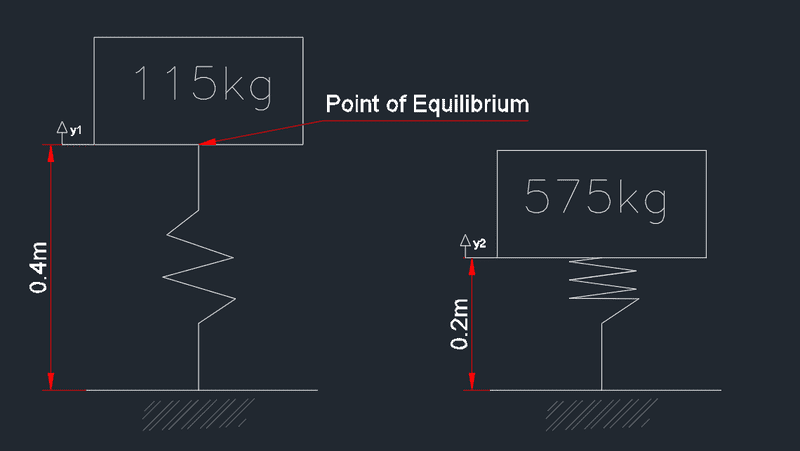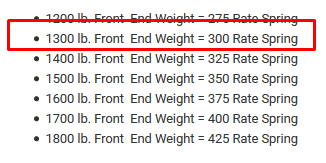# Sizing a spring for a vehicle

## Homework Statement

A mass of 115kg is to be suspended from a spring. When the mass increases to 575kg, the spring will move. Find the spring constant k. (This is not from a text book, it is a project problem for a vehicle unloaded and then loaded on the same spring).
y1 is the maximum height I have but y2 can be changed if needed.(y1-y2)k = mg

## The Attempt at a Solution

(0.2)k = (575)(9.81)
k = 5,640/0.2
k = 28,200N/m

This looks very wrong, comparing to a website that sells a spring holding 1300lb (590kg) gives a rate of 300(136kg/m) or around 1,300N/m

How is my answer so wrong?Last edited:

## Homework Statement

A mass of 115kg is to be suspended from a spring. When the mass increases to 575kg, the spring will move. Find the spring constant k. (This is not from a text book, it is a project problem for a vehicle unloaded and then loaded on the same spring).
y1 is the maximum height I have but y2 can be changed if needed.

View attachment 218411

(y1-y2)k = mg

## The Attempt at a Solution

(0.2)k = (575)(9.81)
k = 5,640/0.2
k = 28,200N/m

This looks very wrong, comparing to a website that sells a spring holding 1300lb (590kg) gives a rate of 300(136kg/m) or around 1,300N/m

How is my answer so wrong?

View attachment 218412
Apologies, the lbs per inch is what put me off. I didn't convert the units properly. However, 300lb/in is = 52kN/m at a 0.3m change in height.

I'm getting

(0.3)k = mg
k = 5,640N/0.3m
k = 18.8kN/m

rude man
Homework Helper
Gold Member
You need to take the difference in x & combine with the difference in weight.That's because you don't know, or haven't given us, the length of the spring when relaxed.